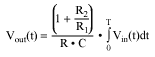# Consider the \"Deboo\" Single-Supply Integrator

### 要約

The \"Deboo\" Integrator simplifies the use of single-supplies by ground-referencing both the input and the output.

The design of standard inverting integrators is simple when bipolar supplies are available, but it's cumbersome with a unipolar supply. To allow adequate headroom for the output, the circuit must be biased away from ground, often at half the supply voltage. Because neither input nor output is referenced to ground, the design is awkward and often impractical.

One solution is a Howland current source with a capacitive load, also known as a Deboo integrator (Figure 1). Powered with bipolar or unipolar supplies, this non-inverting circuit has both a ground-referenced input and output. An intuitive understanding can be gained by considering the circuit in three parts: the input resistor R, the integrating capacitor C, and the right side of the circuit - which is equivalent to a negative resistor connected to ground.Figure 1. The "Deboo integrator" consists of a Howland current source and a capacitive load.

The right side of the circuit (Figure 2a) is easily simplified. Its defining equations areNote that the input current equals the input voltage divided by a negative resistor. The negative resistor just changes the direction of the input current. The corresponding equivalent circuit is inferred by inspection (Figure 2b).Figure 2. In Figure 1, the right-hand side (a) can be simplified to an equivalent circuit (b).

After simplification, the circuit (Figure 3) reveals a "T" configuration, in which Vin drives the capacitor through a positive resistor (R), and ground drives the capacitor through a negative resistor (-R1 × R3/R2).Figure 3. This circuit results from a further simplification of Figure 2b.

Total current into the capacitor isThis important result shows that if R = (R1 × R3)/R2, then current into the capacitor depends only on the input voltage VIN and R and not on the capacitor voltage. This is another way of saying that the capacitor is driven by a current source whose value is VIN/R. If VIN is a function of time, the voltage across the capacitor isVC is then amplified by (1 + R2/R1), so VOUT isThe circuit of Figure 4 is a practical Deboo integrator with two inputs and a reset. The input R is simply the parallel combination of the two 20kΩ resistors. The circuit can sum any number of inputs as long as the parallel combination of the input resistors equals the required R, which in turn equals R1 × R3/R2.Figure 4. This practical implementation of a Deboo integrator has two analog inputs and a reset input.

A single-pole/double-throw analog switch was chosen to perform the reset function. That configuration (vs a single switch across the capacitor) eliminates any reset offset error due to voltage division between the input R and on-resistance in the switch. To prevent the switch from going into its short-circuit-protection mode during reset (which prolongs the reset), voltage across the MAX4624 is limited to less than 0.6V by adding 10Ω in series with the MAX4624. (For capacitors of 0.1µF or less, this voltage-limiting resistor is unnecessary.)

Many parameters should be considered when designing an integrator. Fast integrators require wideband op amps with low bias current. Very slow integrators require closely matched resistors, op amps with extremely low bias current, capacitors with low leakage current, and a physically clean board layout. Polypropylene, polystyrene, or teflon are the dielectrics of choice for capacitors with low leakage and low dielectric absorption.

To implement a single-input integrator, the four resistors can have the same value. Quad equal-value resistor packs with ratio tolerances down to ±0.01% are available from Caddock Electronics, but less demanding requirements can allow the use of four discrete ±1% resistors. Choose the reset switch carefully, because its off-leakage is integrated by the capacitor. The switch on-resistance must be low enough to allow the capacitor to be reset as quickly as necessary. Finally, charge injected by the switch during turn off must be sufficiently low to avoid an objectionably large offset on the capacitor.

The Deboo integrator is easily implemented and very useful, particularly for single-supply applications.